Next: Further Investigation Up: The Chaotic Pendulum Previous: Definition of Chaos

Periodic Windows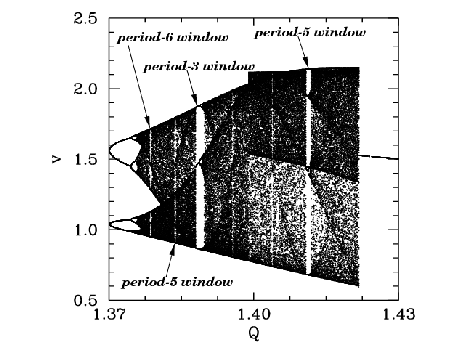Let us return to Figure 78. Recall, that this figure shows the onset of chaos, via a cascade of period-doubling bifurcations, as the quality-factor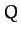is gradually increased. Figure 87 is essentially a continuation of Figure 78 which shows the full extent of the chaotic region (in-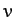space). It can be seen that the chaotic region ends abruptly whenexceeds a critical value, which is about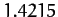. Beyond this critical value, the time-asymptotic motion appears to revert to period-1 motion (i.e., the solid black region collapses to a single curve). It can also be seen that the chaotic region contains many narrow windows in which chaos reverts to periodic motion (i.e., the solid black region collapses tocurves, whereis the period of the motion) for a short interval in. The four widest windows are indicated in the figure.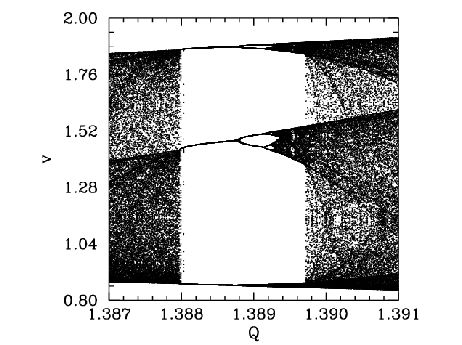Figure 88 is a blow-up of the period-3 window shown in Figure 87. It can be seen that the window appears out of the blue'' asis gradually increased. However, it can also be seen that, asis further increased, the window breaks down, and eventually disappears, due to the action of a cascade of period-doubling bifurcations. The same basic mechanism operates here as in the original period-doubling cascade, discussed in Section 15.9, except that now the orbits are of period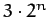, instead of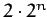. Note that all of the other periodic windows seen in Figure 87 break down in an analogous manner, asis increased.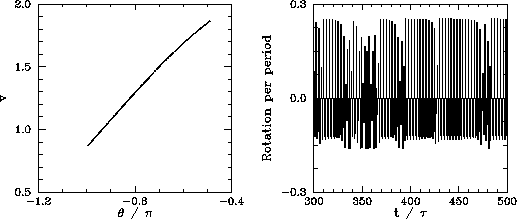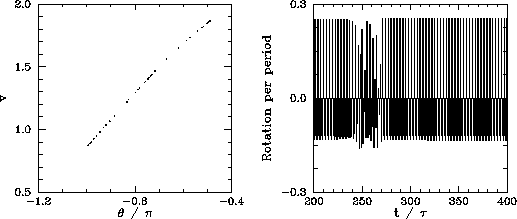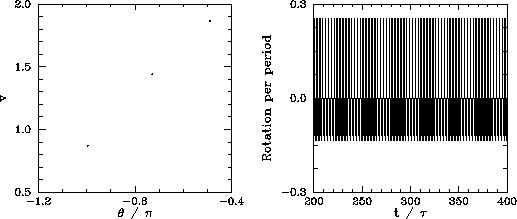We now understand how periodic windows break down. But, how do they appear in the first place? Figures 89-91 show details of the pendulum's time-asymptotic motion calculated just before the appearance of the period-3 window (shown in Figure 88), just at the appearance of the window, and just after the appearance of the window, respectively. It can be seen, from Figure 89, that just before the appearance of the window the attractor is chaotic (i.e., its Poincaré section consists of a line, rather than a discrete set of points), and the time-asymptotic motion of the pendulum consists of intervals of period-3 motion interspersed with intervals of chaotic motion. Figure 90 shows that just at the appearance of the window the attractor loses much of its chaotic nature (i.e., its Poincaré section breaks up into a series of points), and the chaotic intervals become shorter and much less frequent. Finally, Figure 91 shows that just after the appearance of the window the attractor collapses to a period-3 attractor, and the chaotic intervals cease altogether. All of the other periodic windows seen in Figure 87 appear in an analogous manner to that just described.

According to the above discussion, the typical time-asymptotic motion seen just prior to the appearance of a period-window consists of intervals of period-motion interspersed with intervals of chaotic motion. This type of behaviour is called intermittency, and is observed in a wide variety of non-linear systems. As we move away from the window, in parameter space, the intervals of periodic motion become gradually shorter and more infrequent. Eventually, they cease altogether. Likewise, as we move towards the window, the intervals of periodic motion become gradually longer and more frequent. Eventually, the whole motion becomes periodic.

In 1973, Metropolis and co-workers investigated a class of simple mathematical models which all exhibit a transition to chaos, via a cascade of period-doubling bifurcations, as some control parameter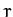is increased.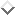They were able to demonstrate that, for these models, the order in which stable periodic orbits occur asis increased is fixed. That is, stable periodic attractors always occur in the same sequence asis varied. This sequence is called the universal or U-sequence. It is possible to make a fairly convincing argument that any physical system which exhibits a transition to chaos via a sequence of period-doubling bifurcations should also exhibit the U-sequence of stable periodic attractors. Up to period-6, the U-sequence is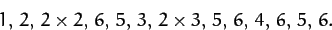The beginning of this sequence is familiar: periods 1, 2,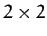are the first stages of the period-doubling cascade. (The later period-doublings give rise to periods greater than 6, and so are omitted here). The next periods,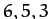correspond to the first three periodic windows shown in Figure 87. Period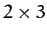is the first component of the period-doubling cascade which breaks up the period-3 window. The next period, 5, corresponds to the last periodic window shown in Figure 87. The remaining periods, 6, 4, 6, 5, 6, correspond to tiny periodic windows, which, in practice, are virtually impossible to observe. It follows that our driven pendulum system exhibits the U-sequence of stable periodic orbits fairly convincingly. This sequence has also been observed experimentally in other, quite different, dynamical systems.The existence of a universal sequence of stable periodic orbits in dynamical systems which exhibit a transition to chaos via a cascade of period-doubling bifurcations is another indication that chaos is a universal phenomenon.Next: Further Investigation Up: The Chaotic Pendulum Previous: Definition of Chaos
Richard Fitzpatrick 2011-03-31